Connect to our Experts within seconds for a free diagnosis
Our professional experts are available now. Your privacy is guaranteed.

# Excel AVERAGE Function

The average of a set of numbers is calculated by adding up the numbers, then dividing this sum by the count of numbers that are being averaged. On this same approach, we can calculate the average of a set of numbers by using the Excel AVERAGE function.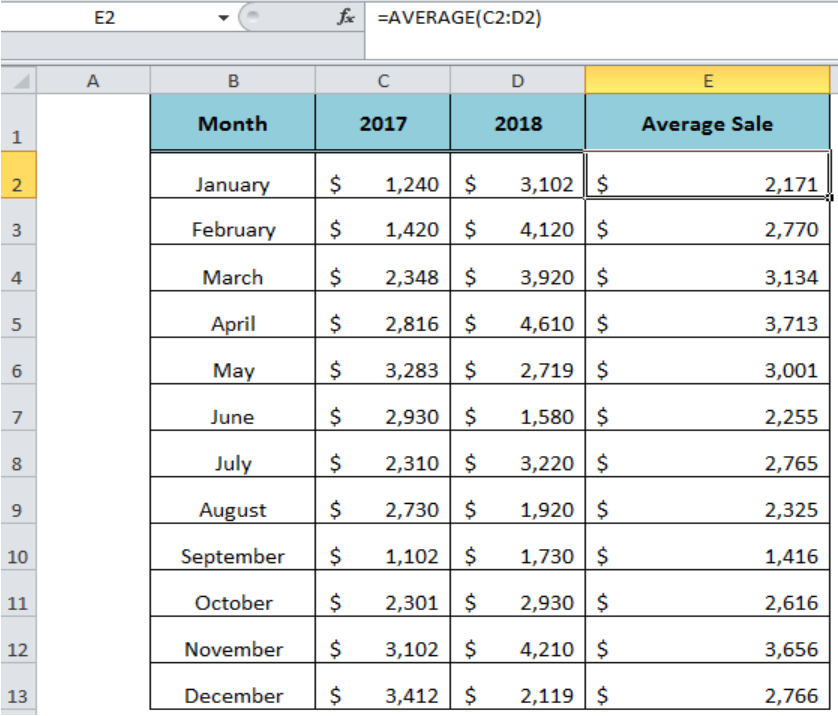Figure 1. Using the Excel AVERAGE Function

## Syntax

The syntax for the AVERAGE function is;

`=AVERAGE (number1, [number2], ...)`

Where,

• Number1 – It is a required argument and can be entered as a number, cell reference, range, array or a mix of these. The function ignores the empty cell.
• Number2 – It is optional to add more numbers up to 255 arguments in total.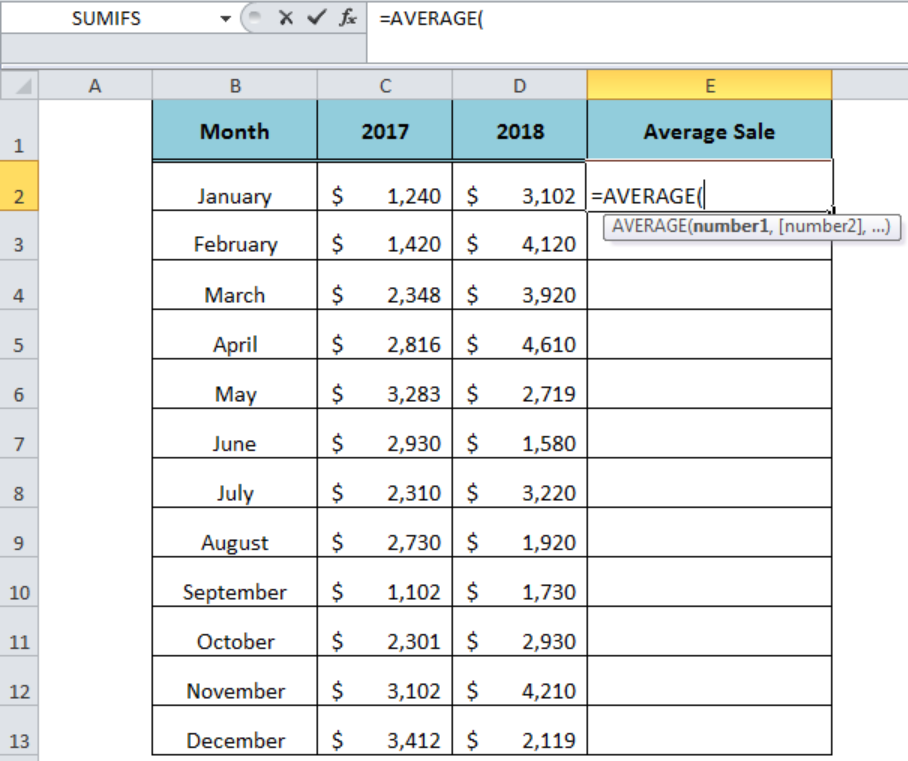Figure 2. The Syntax For the AVERAGE Function

## Using the Cell Reference

We can supply the arguments in the AVERAGE function as cell reference or directly as a constant number. To calculate the average sales for 2017 and 2018 we can supply the cell references, such as;

`=AVERAGE(C2,D2)`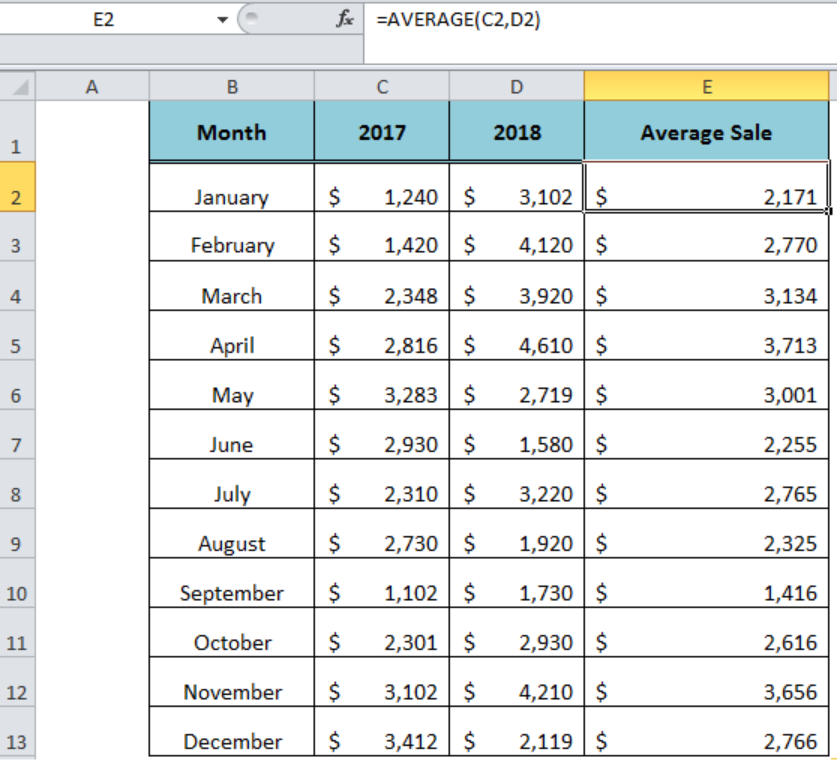Figure 3. Using the Cell References in AVERAGE Function

## Using the Range

The range consists of cells in one row or one column. We can supply the range of cells containing the numeric values as the argument of the AVERAGE function to return the result, such as;

`=AVERAGE(C2: D2)`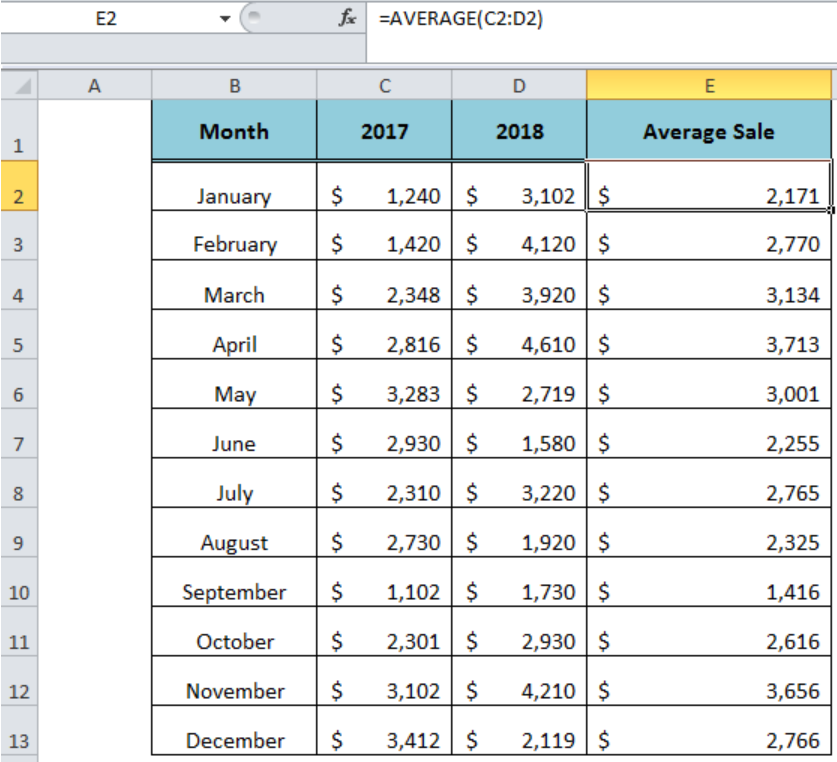Figure 4. Using the Range in AVERAGE Function

## Using the Array

An array contains cells of multiple columns and rows. We can calculate the average of numbers by entering the array as an argument in the AVERAGE function. To calculate the average sales of both 2017 and 2018, we need to enter the array in the function, such as;

`=AVERAGE(C2: D13)`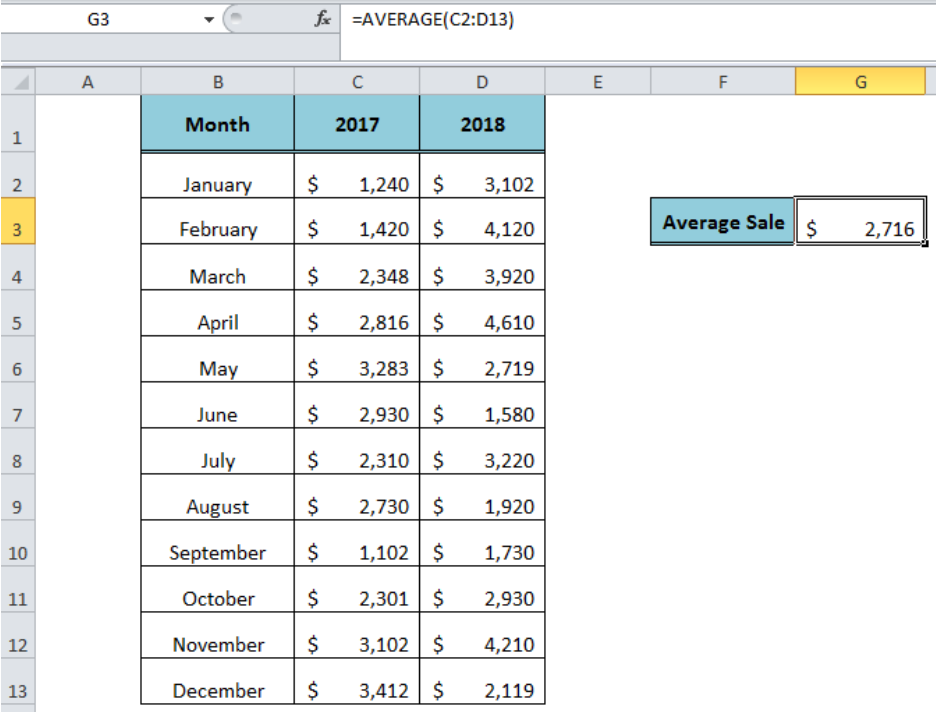Figure 5. Using the Array in AVERAGE Function

## Instant Connection to an Expert through our Excelchat Service:

Most of the time, the problem you will need to solve will be more complex than a simple application of a formula or function. If you want to save hours of research and frustration, try our live Excelchat service! Our Excel Experts are available 24/7 to answer any Excel question you may have. We guarantee a connection within 30 seconds and a customized solution within 20 minutes.

### Did this post not answer your question? Get a solution from connecting with the expert.Another blog reader asked this question today on Excelchat:Another blog reader asked this question today on Excelchat: